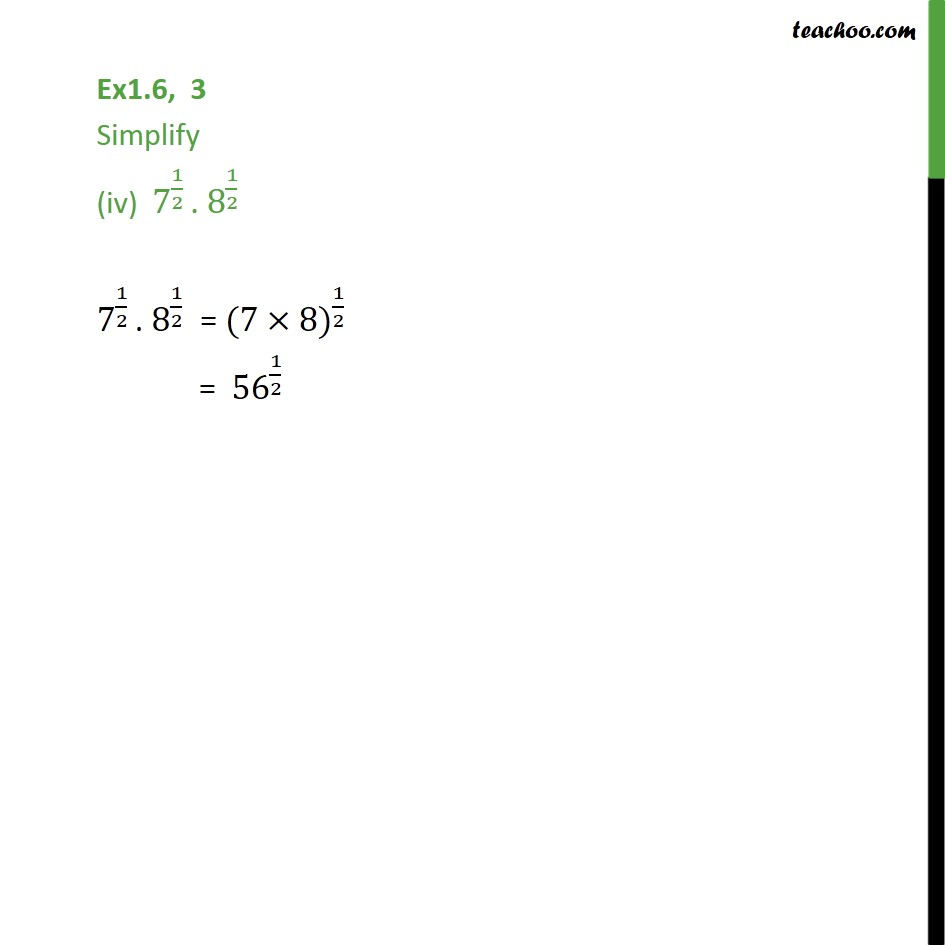1. Chapter 1 Class 9 Number Systems (Term 1)
2. Serial order wise
3. Ex 1.6

Transcript

Ex1.6, 3 Simplify (iv) 7^(1/2) . 8^(1/2) 7^(1/2) . 8^(1/2) = (7 8) ^(1/2) = 56 ^(1/2)